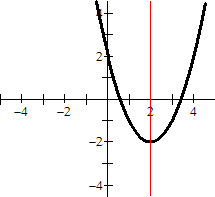index: click on a letter A B C D E F G H I J K L M N O P Q R S T U V W X Y Z A to Z index index: subject areas numbers & symbols sets, logic, proofs geometry algebra trigonometry advanced algebra & pre-calculus calculus advanced topics probability & statistics real world applications multimedia entrieswww.mathwords.com about mathwords website feedback

Axis of Symmetry

A line of symmetry for a graph. The two sides of a graph on either side of the axis of symmetry look like mirror images of each other.

 Example:This is a graph of the parabola y = x2 – 4x + 2 together with its axis of symmetry x = 2. The axis of symmetry is the red vertical line.